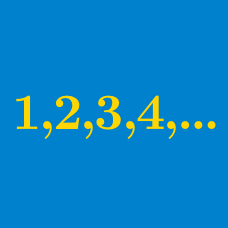Algebra

# Arithmetic Progression Comparisons

Mary and Tina are hired for a new job with a base salary of $30,000 per year. Each year, Mary receives a$2,000 raise, while Tina only receives a $500 raise. After 5 years have elapsed (so that each of them has received 5 raises), how much greater is Mary’s yearly salary than Tina’s? On Day 1 starting at a new job, John is paid$20 and Jack is paid $32. Every subsequent day, John is paid$3 more than the previous day, while Jack is paid $1 more than the previous day. On which day will they be paid the same amount of money? $$\{a_n\}$$ is an arithmetic progression whose terms are $$a_1, a_2, a_3, …$$ $$\{b_n\}$$ is an arithmetic progression whose terms are $$b_1, b_2, b_3, …$$ If a new sequence $$\{a_1 +b_1, a_2 + b_2, a_3 + b_3, …\}$$ is made by adding the terms of $$\{a_n\}$$ and $$\{b_n\}$$, is the new sequence an arithmetic progression? $$\{a_n\}$$ is an arithmetic progression whose terms are $$a_1, a_2, a_3, ...$$ and whose common difference is $$d = a_2 - a_1.$$ $$\{b_n\}$$ is an arithmetic progression created by multiplying each term in $$\{a_n\}$$ by 2. $$\{c_n\}$$ is an arithmetic progression whose first term is the same as $$\{a_n\}$$ and whose common difference is twice the common difference of $$\{a_n\}.$$ What is the value of $$b_{10} - c_{10}?$$ Tom and Sarah start a new job. On Day 1, Tom is paid$20 and Sarah is paid $40. Each subsequent day, Tom is paid$5 more than the previous day, while Sarah is paid \$3 more than the previous day.

At the end of Day 15, who has made a greater total amount of money over the course of the whole job?

×